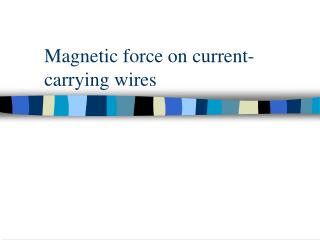DownloadDownload PresentationMagnetic force on current-carrying wires

# Magnetic force on current-carrying wires

Télécharger la présentation## Magnetic force on current-carrying wires

- - - - - - - - - - - - - - - - - - - - - - - - - - - E N D - - - - - - - - - - - - - - - - - - - - - - - - - - -
##### Presentation Transcript

1. Magnetic force on current-carrying wires

2. Warm-up 1 In 16.2, we learnt that a fixed current-carrying wire deflects a movable compass needle (Fig a). What happens if a current flows along a movable wire between fixed magnets (Fig b)? Fig a A The wire moves. B The wire does not move. C It may or may not move. It cannot be predicted. Fig b

3. Warm-up 2 Below shows a side-view of: Try to draw the magnetic field lines of (a) the magnet, • (b) the wire. What happens when the field lines from the magnet and the wire 'cross' each other.

4. Introduction If a current flows in a conductor and the conductor makes an angle with magnetic field lines, then a force acts on the conductor. This explains why a loudspeaker paper cone vibrates and an electric motor rotates. We can do an experiment to find out about the force on a current-carrying conductor.

5. Experiment 16d Force on a current-carrying conductor Investigate the force acting on a current-carrying wire in a magnetic field. slab-shaped magnets on steel yoke to low-voltage power supply

6. 1 Force on a current-carrying conductor force When wire carries a current I, I wire is moved upwards by an upward force. I If the direction of either I or B-field is reversed, wire is moved downwards by a downward force.

7. 1 Force on a current-carrying conductor force How to determine the direction of force on the current-carrying wire? I I

8. Fleming’s left-hand rule: F magnetic field B force F Hold the thumb, firstand second finger of LEFT HANDat right angles. B current, I I This is a rule to help you to remember their directions. (NOT a physics principle!)

9. Fleming’s left-hand rule: Remarks: (1) If current & field NOT at right angles,  force (2) If current & field parallel,  NOforce (3) Force if • I  • use stronger magnet • length of wire inside magnetic field 

10. Combined magnetic field 10-turn coil of enamelled copper wire demonstration meter 05 A slab-shaped magnets This apparatus is used to show 1 circular field lines due to I in a straight wire 2 straight field lines of 2 slab-shaped magnets 3 the combined field of (1) & (2) low voltage supply plastic plate

11. +  Combined magnetic field Results: It appears: the wire is being ‘catapulted’ in a direction from strong field region to week field region. = neutral point

12. Moving-coil loudspeakers

13. Moving-coil loudspeakers Contains a short-coil: free to move inside a cylindrical permanent magnet. stiff paper or plastic cone circular pole coil radial magnetic field short coil Wirefield

14. Moving-coil loudspeakers As a.c. passes through the coil, Iflows backwards & forwards circular pole coil stiff paper or plastic cone radial magnetic field short coil  coil is pushed in & out gives out sound waves

15. Example 4 Interaction between current-carrying wires 2 parallel wires A & B, carry the same size of current. • • A B (a) Draw a few magnetic field lines due to the current through wire A.

16. Example 4 Interaction between current-carrying wires 2 parallel wires A & B, carry the same size of current. field at Bdue to current through A • • A B (b) Mark the direction of this magnetic field at wire B with an arrow.

17. Example 4 Interaction between current-carrying wires 2 parallel wires A & B, carry the same size of current. magnetic force field at Bdue to current through A • • A B (c) Mark the direction of magnetic force acting on B due to magnetic field of the current through A.

18. Example 4 Interaction between current-carrying wires 2 parallel wires A & B, carry the same size of current. magnetic force field at Bdue to current through A • • field at Adue to current through B A B (d) Deduce the direction of magnetic force acting on A due to magnetic field of the current through B.

19. Example 4 Interaction between current-carrying wires 2 parallel wires A & B, carry same size of current. magnetic force field at Bdue to current through A • • field at Adue to current through B A B (e) Do A & B attract or repel each other? They attract each other.

20. Q1 What is the direction of... What is the direction of the magnetic force acting on the wire MN and OP? O N P M Segment MN Segment OP A upwards upwards B downwards downwards C upwards downwards D downwards upwards

21. Q2 Describe the direction of... Describe the direction of magnetic force acting on the conductor AB. A B The current is parallel to the magnetic field and no magnetic force acts on AB.

22. A B Q3 Redraw the diagram so that... Redraw the diagram so that the magnetic force acting on the conductor AB is greatest and pointing out of the paper. B A

23. Q4 A magnetic force acts on the... A magnetic force acts on the current-carrying metal rider and makes the rider move (see Experiment 16d). Does the magnetic force do work on the rider? (Yes / No)

24. Q5 A magnet exerts a force on... A magnet exerts a force on a current-carrying wire. For two parallel current-carrying wires, does one of them exert a force on the other? (Yes / No)